# Prime Numbers 1 to 100

Prime Numbers hold a significant place in the evolution of mathematics, primarily for their crucial role in improving cryptography and security standards in the modern world. Despite years of research, Prime Numbers continue to be an active topic in the field of mathematical research, fascinating scientists and scholars with their elegant properties and wide range of applications.

In this article, you will read about what are prime numbers, prime numbers 1 to 100, list of prime numbers, differences between prime numbers and composite numbers, examples of prime numbers, etc.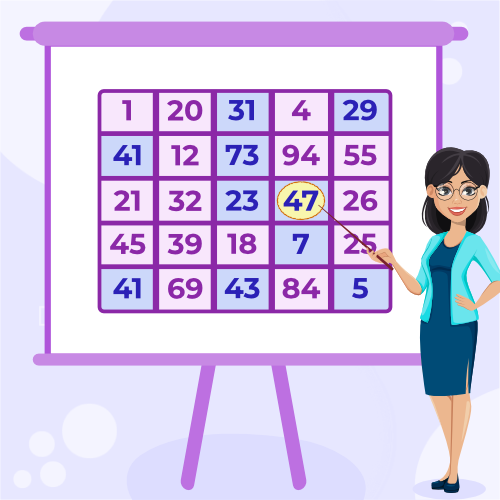## What are Prime Numbers in Maths?

Prime Numbers are those natural numbers whose only divisors are 1 and the number itself. If a number p is prime, then its only possible factors are 1 and p itself, there should not be any other factors of p if p is prime. Some examples of primes are 2, 3, 5, 7, 11, 13, 31, 79, 19, 239, 137, 419, 191, 409, etc. Also, the smallest Prime Number is 2.

### Prime Numbers Definition

A prime number is a whole number greater than 1 that is divisible only by 1 and itself.

## History of Prime Numbers

Throughout history, prime numbers have been considered among the most fascinating numbers in mathematics. From ancient Greeks to modern-day cryptographers, people have discussed primes with enthusiasm. Many scientists, philosophers, and mathematicians have worked and researched on primes throughout history, including Euclid, Eratosthenes, al-Khwarizmi (an Arab mathematician), Qin Jiushao (a Chinese mathematician), Leonardo Fibonacci, Pierre de Fermat, Marin Mersenne, and many others.

## Prime Numbers List

There are infinitely many Prime Numbers and that is proven by the great philosopher and mathematician Euclid (father of geometry) in the year 300 BC.

### List of Prime Numbers from 1 to 100

There are 25 prime numbers between 1 to 100 –

2, 3, 5, 7, 11, 13, 17, 19, 23, 29, 31, 37, 41, 43, 47, 53, 59, 61, 67, 71, 73, 79, 83, 89, 97

The image added below shows the prime numbers from 1 to 100.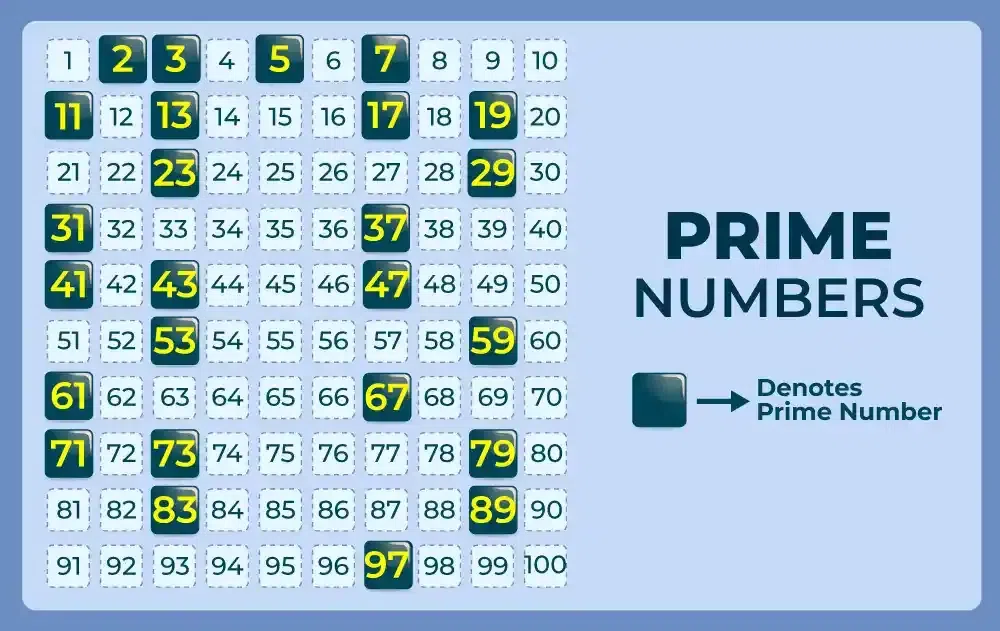### Prime Numbers from 1 to 50

The list of Prime Numbers between 1 and 50 is as follows:

2, 3, 5, 7, 11, 13, 17, 19, 23, 29, 31, 37, 41, 43, 47

There are 15 prime numbers between 1 and 50.

### Prime Numbers from 1 to 200

The list of Prime Numbers between 1 and 200 is as follows:

2, 3, 5, 7, 11, 13, 17, 19, 23, 29, 31, 37, 41, 43, 47, 53, 59, 61, 67, 71, 73, 79, 83, 89, 97, 101, 103, 107, 109, 113, 127, 131, 137, 139, 149, 151, 157, 163, 167, 173, 179, 181, 191, 193, 197, 199

There are 46 prime numbers between 1 and 200.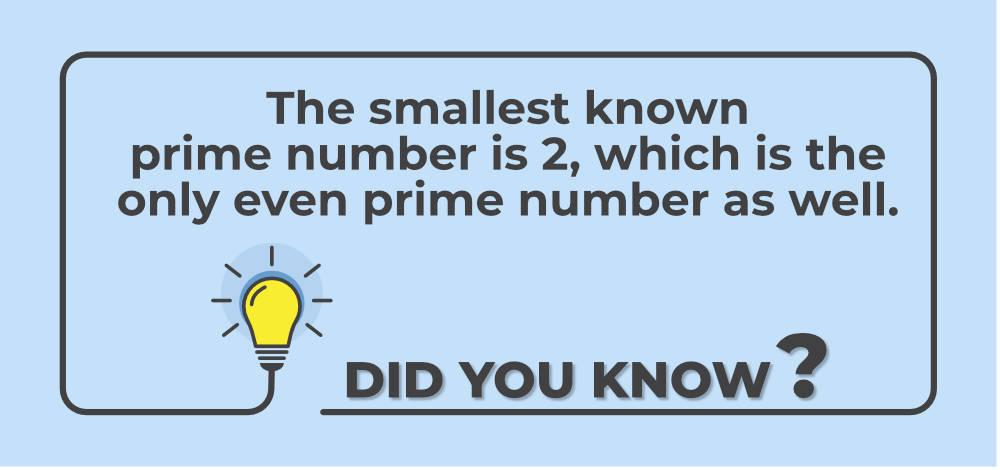## Prime Numbers 1 to 1000

There are 168 prime numbers between 1 and 1000. List of Prime Numbers between 1 and 1000 are:

2, 3, 5, 7, 11, 13, 17, 19, 23, 29, 31, 37, 41, 43, 47, 53, 59, 61, 67, 71, 73, 79, 83, 89, 97, 101, 103, 107, 109, 113, 127, 131, 137, 139, 149, 151, 157, 163, 167, 173, 179, 181, 191, 193, 197, 199, 211, 223, 227, 229, 233, 239, 241, 251, 257, 263, 269, 271, 277, 281, 283, 293, 307, 311, 313, 317, 331, 337, 347, 349, 353, 359, 367, 373, 379, 383, 389, 397, 401, 409, 419, 421, 431, 433, 439, 443, 449, 457, 461, 463, 467, 479, 487, 491, 499, 503, 509, 521, 523, 541, 547, 557, 563, 569, 571, 577, 587, 593, 599, 601, 607, 613, 617, 619, 631, 641, 643, 647, 653, 659, 661, 673, 677, 683, 691, 701, 709, 719, 727, 733, 739, 743, 751, 757, 761, 769, 773, 787, 797, 809, 811, 821, 823, 827, 829, 839, 853, 857, 859, 863, 877, 881, 883, 887, 907, 911, 919, 929, 937, 941, 947, 953, 967, 971, 977, 983, 991, 997

### List of Odd Prime Numbers

As 2 is the only even prime number, the list of the first 100 odd prime numbers is the same as the list of the first 101 prime numbers without the first prime 2.

3, 5, 7, 11, 13, 17, 19, 23, 29, 31, 37, 41, 43, 47, 53, 59, 61, 67, 71, 73, 79, 83, 89, 97, 101, 103, 107, 109, 113, 127, 131, 137, 139, 149, 151, 157, 163, 167, 173, 179, 181, 191, 193, 197, 199, 211, 223, 227, 229, 233, 239, 241, 251, 257, 263, 269, 271, 277, 281, 283, 293, 307, 311, 313, 317, 331, 337, 347, 349, 353, 359, 367, 373, 379, 383, 389, 397, 401, 409, 419, 421, 431, 433, 439, 443, 449, 457, 461, 463, 467, 479, 487, 491, 499, 503, 509, 521, 523, 541

### Even Prime Number

2 is the only even prime number in the list of infinitely many primes as all the other even numbers have one factor as 2. For example, take any random even number i.e., 2k for some k, now as 2k is always divisible by 2. Thus it has 2 as its factor. Thus no even number can be prime except 2.

### Smallest Prime Number

As primes are defined as a subset of natural numbers so the possibility of the smallest prime can’t be less than 1 but 1 is not a prime number, thus the second natural number i.e., 2 is considered the smallest prime number.

### Largest Prime Number

There are much larger Prime Numbers which is discovered and the method which is used to discover the larger primes is GIMPS(Great Internet Mersenne Prime Search) [ a prime number of the form 2p -1, is called Mersenne Primes]. The largest prime discovered by this method is 282,589,933 -1, which was discovered by a person named Patrick Laroche.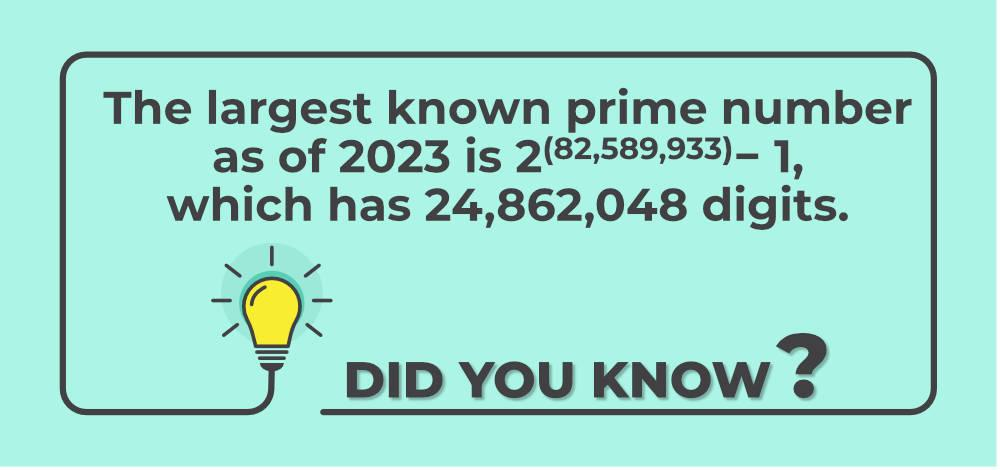## How to Find Prime Numbers?

One of the easiest methods to determine whether a number is prime is to divide it by integers from 2 up to the square root of the number. If none of these divisions results in an integer, then the number is prime. While there are other methods to find primes, such as the Miller-Rabin Primality Test and AKS Primality Test, most of them require significant computing power to check the prime nature of very large numbers. Primes are widely used in the fields of cryptography, number theory, and computer science.

## Properties of Prime Numbers

Prime numbers have some properties which are listed as follows:

• A Prime number is always a natural number i.e., a number greater than 1.
• The first few prime numbers are 2, 3, 5, 7, 11, 13, 17, 19, 23, 29, 31, 37, 41, 43, 47, and so on.
• There are infinitely many prime numbers. This was proved by Euclid around 300 BC.
• Every positive integer greater than 1 can be written as a product of prime numbers in a unique way, up to the order of the factors. This is known as the Fundamental Theorem of Arithmetic.
• If p is a prime number such that p | ab (Where a and b are two integers), then p must divide at least one of them and this is known as Euclid’s lemma.
• According to Fermat’s Little theorem, if p is a prime number and a is a positive integer not divisible by p, then there exists a positive integer k such that ak – 1 is divisible by p.

## Composite Numbers

The number which is not prime except 1 are called composite numbers. In other words, if for any number a, there are factors other than 1 and itself then a is called a composite number. For example, 4 as it has 2 as its factor other than 1 and 4. So 4 is a composite number. Some more examples of composite numbers are 6, 8, 9, 12, 14, 15, etc.

## Prime Numbers Vs Composite Numbers

There are a few differences between Prime and Composite numbers, which are as follows:

Prime Numbers

Composite Numbers

All Prime Numbers have only two factors such as 1 and the number itself. For example, 5 has only two factors 1 and 5. All Composites Numbers must have any factor other than 1 and the number itself. For example, 6 has 2 and 3 as its factors other than 1 and 6.
All Primes are Odd numbers except 2. Composites can be either odd or even.
Some of the first few primes are 2, 3, 5, 7, 11, 13, 17, etc. Some of the first few composites are 4, 6, 8, 9, 12, 14, 15, etc.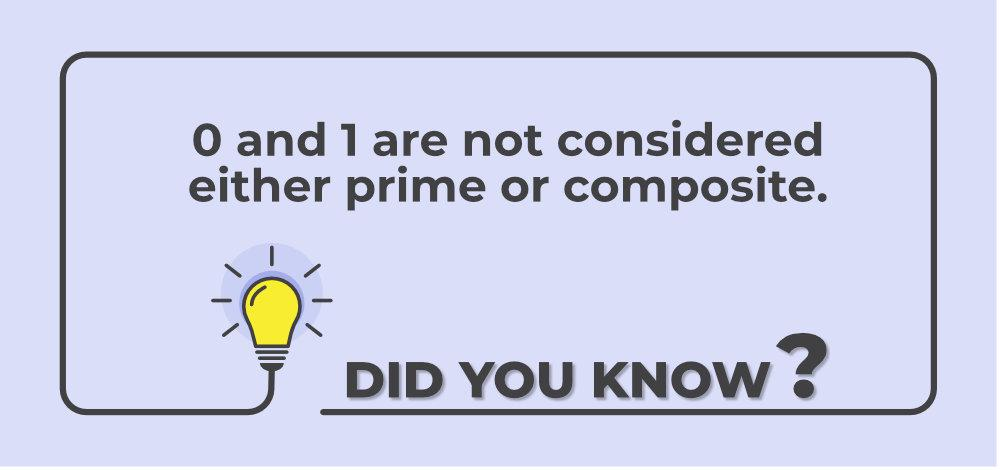## Coprime Numbers

For any two numbers (need not be prime themselves) to be coprime there is only one condition that needs to be satisfied i.e. the highest common factor of both numbers is to be 1. For example, 8 and 15 are coprime, as factors of 8 excluding 1 and 8 are 2, 4 and factors of 15 excluding 1 and 15 are 3 and 5, so only common factors for 8 and 15 are 1.

Some more examples of Coprime pairs are 4 and 7, 10 and 13, 22 and 25, etc.

Note: All primes numbers are coprime to each other.

Resources related to Prime Numbers:

## Solved Examples on Prime Numbers

Example 1: Determine whether 37 is a prime number or not.

Solution:

As a prime number is a positive integer greater than 1 that has no positive integer divisors other than 1 and itself.

As 37 = 1 × 37,

Therefore, 37 has no divisors other than 1 and itself, and it is a prime number.

So, 37 is a prime number.

Example 2: Find all the prime numbers between 20 and 40.

Solution:

• 20 is not a prime number, since it is divisible by 2.
• 21 is not a prime number, since it is divisible by 3.
• 22 is not a prime number, since it is divisible by 2.
• 23 is a prime number since it has no divisors other than 1 and itself.
• 24 is not a prime number, since it is divisible by 2.
• 25 is not a prime number, since it is divisible by 5.
• 26 is not a prime number, since it is divisible by 2.
• 27 is not a prime number, since it is divisible by 3.
• 28 is not a prime number, since it is divisible by 2.
• 29 is a prime number since it has no divisors other than 1 and itself.
• 30 is not a prime number, since it is divisible by 2 and 3.
• 31 is a prime number since it has no divisors other than 1 and itself.
• 32 is not a prime number, since it is divisible by 2.
• 33 is not a prime number, since it is divisible by 3.
• 34 is not a prime number, since it is divisible by 2.
• 35 is not a prime number, since it is divisible by 5.
• 36 is not a prime number, since it is divisible by 2 and 3.
• 37 is a prime number since it has no divisors other than 1 and itself.
• 38 is not a prime number, since it is divisible by 2.
• 39 is not a prime number, since it is divisible by 3.
• 40 is not a prime number, since it is divisible by 2 and 5.

Therefore, the prime numbers between 20 and 40 are 23, 29, and 37.

## Practice Problems on Prime Numbers

1. Find the first 5 prime numbers.

2. Determine whether the following numbers are prime or not: 17, 25, 29, 37, 41.

3. Calculate the product of the first 3 prime numbers.

4. What is the sum of the first 10 prime numbers?

5. Find the prime factorization of the number 56.

## Prime Numbers – FAQs

### 1. What are Prime numbers in math?

Prime numbers are positive integers greater than 1 that has no positive integer divisors other than 1 and itself.

### 2. What prime numbers are even?

There is only one such prime number which is even i.e. 2. Therefore 2 is called only even prime.

### 3. Is 1 a prime number?

1 is neither prime nor composite number.

### 4. What are twin prime numbers?

Twin prime are the pair of primes which has difference of 2(when smaller is subtracted from greater). For example 5 and 7 as 7-5=2.

Some more examples of twin primes are 3 and 5 (5-3=2),11 and 13, 17 and 19, 29 and 31, 41 and 43, etc.

### 5. What are the first 10 prime numbers?

First ten primes are 2, 3, 5, 7, 11, 13, 17, 19, 23 and 29.

### 6. What are Twisted Prime Numbers?

A pair of number is called Twisted Primes if both numbers are palindrome as well as prime themselves. For example, 17 and 71 are Twisted Prime Pair as both are pallendrom (reversing the digits of 17 number become 71 and same for the 71) as well as prime themselves.

### 7. Why is 2 a Prime Number?

2 is considered a prime number because it meets the fundamental definition of a prime number: it is a positive integer greater than 1 that has no positive integer divisors other than 1 and itself. In the case of 2, it can only be divided by 1 and 2 without leaving a remainder.

Whether you're preparing for your first job interview or aiming to upskill in this ever-evolving tech landscape, GeeksforGeeks Courses are your key to success. We provide top-quality content at affordable prices, all geared towards accelerating your growth in a time-bound manner. Join the millions we've already empowered, and we're here to do the same for you. Don't miss out - check it out now!

Previous
Next